# Principal Nested Spheres Backwards PCA Backwards PCA Backwards

• Slides: 78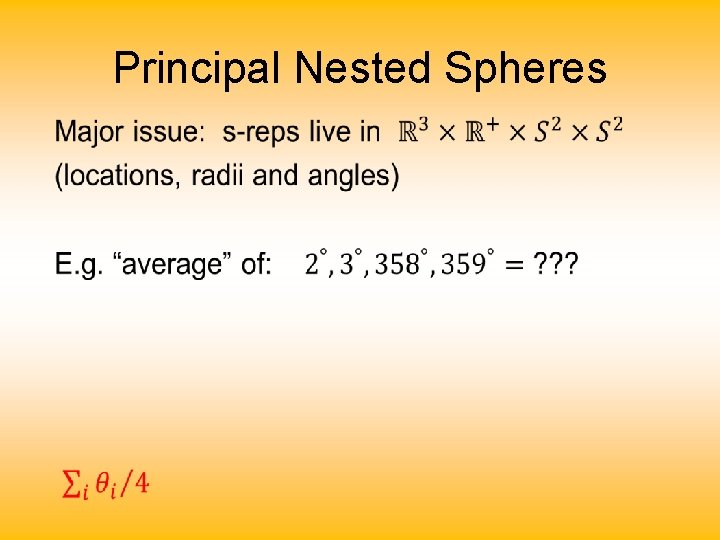Principal Nested Spheres •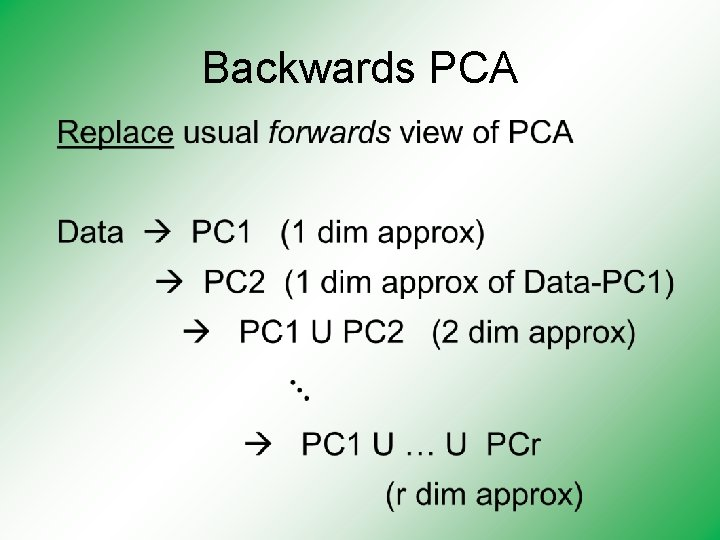Backwards PCA •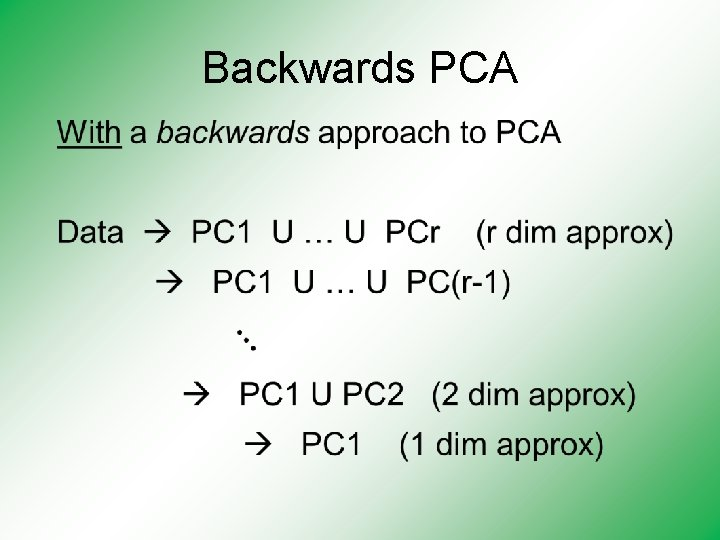Backwards PCA •Backwards PCA Euclidean Settings: Forwards PCA = Backwards PCA (Pythagorean Theorem, ANOVA Decomposition) So Not Interesting But Very Different in Non-Euclidean Settings (Backwards is Better !? !)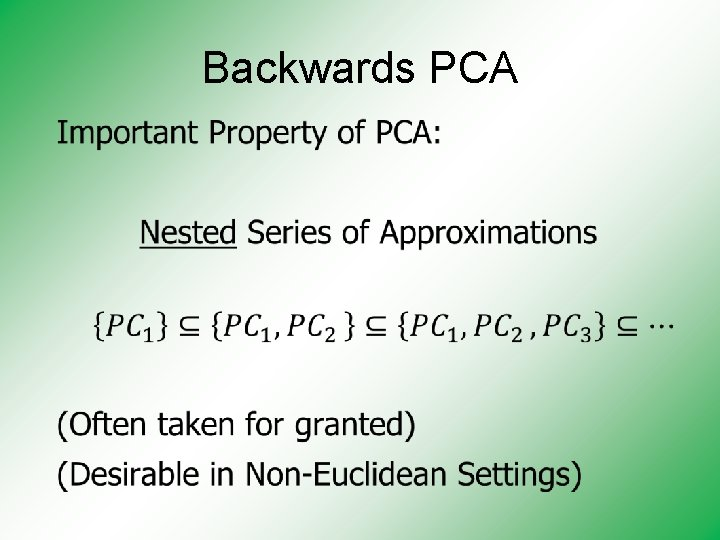Backwards PCA •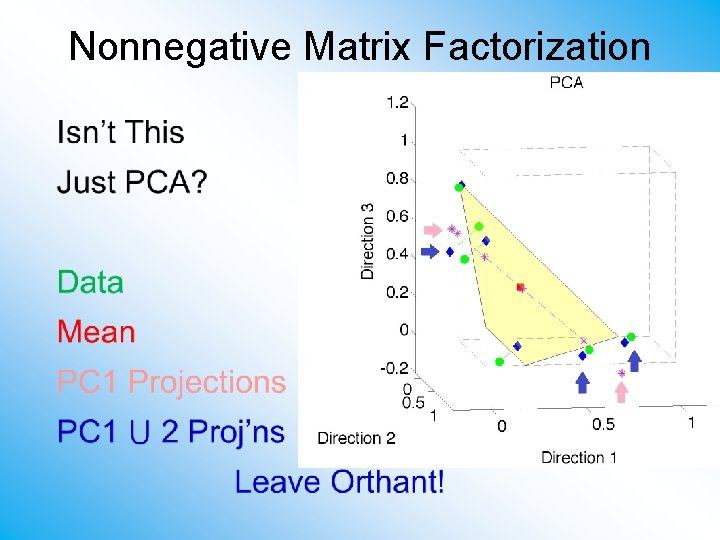Nonnegative Matrix Factorization •Nonnegative Matrix Factorization Standard NMF (Projections All Inside Orthant)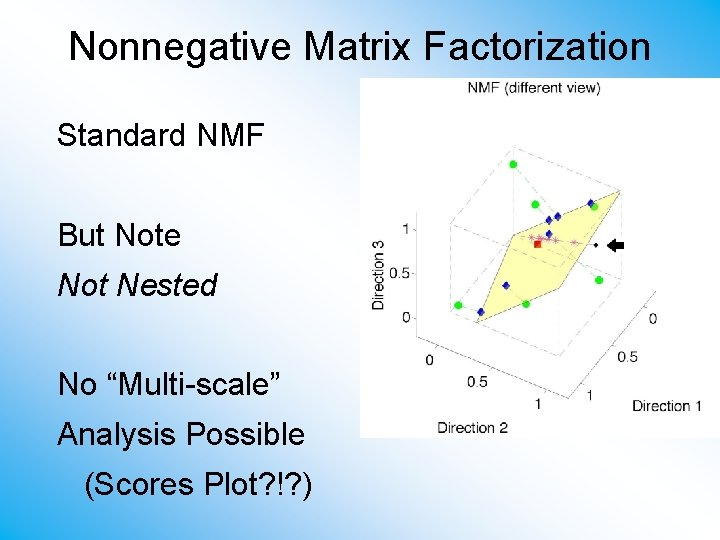Nonnegative Matrix Factorization Standard NMF But Note Not Nested No “Multi-scale” Analysis Possible (Scores Plot? !? )Nonnegative Nested Cone Analysis Same Toy Data Set All Projections In OrthantNonnegative Nested Cone Analysis Same Toy Data Set Rank 1 Approx. Properly Nested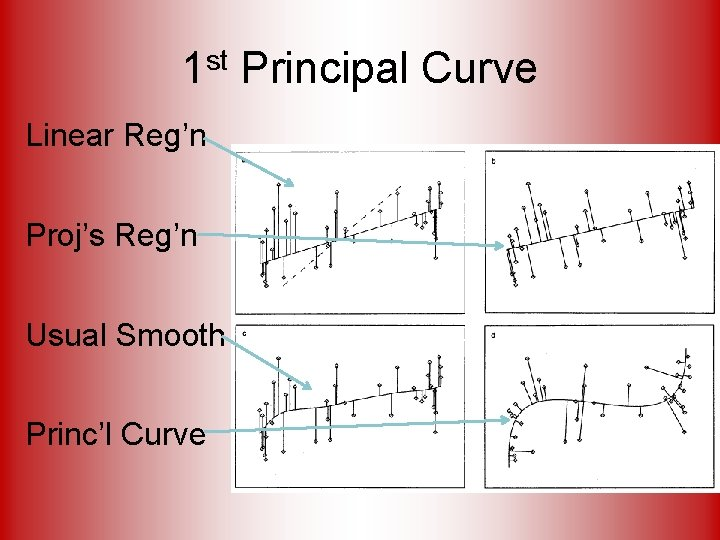1 st Principal Curve Linear Reg’n Proj’s Reg’n Usual Smooth Princ’l CurveManifold Learning How generally applicable is Backwards approach to PCA? Potential Application: Principal Curves Perceived Major Challenge: How to find 2 nd Principal Curve?Manifold Learning Key Component: Principal Surfaces Le. Blanc & Tibshirani (1996) Challenge: Can have any dimensional surface, But how to nest? ? ? Proposal: Backwards ApproachAn Interesting Question How generally applicable is Backwards approach to PCA? An Attractive Answer: James Damon, UNC Mathematics Key Idea: Express Backwards PCA as Nested Series of Constraints• General View of Backwards PCAGeneral View of Backwards PCA Define Nested Spaces via Constraints • Backwards PCA • Principal Nested Spheres • Principal Surfaces • Other Manifold Data Spaces Sub-Manifold Constraints? ? (Algebraic Geometry)Detailed Look at PCA Now Study “Folklore” More Carefully • Back. Ground • History • Underpinnings (Mathematical & Computational) Good Overall Reference: Jolliffe (2002)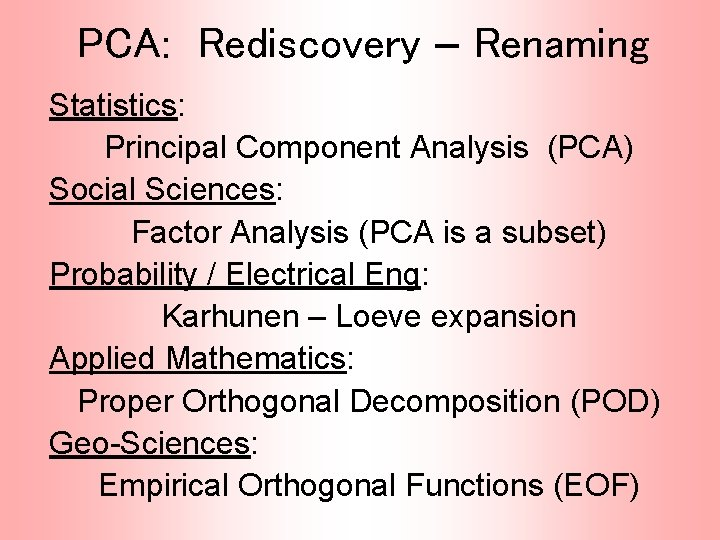PCA: Rediscovery – Renaming Statistics: Principal Component Analysis (PCA) Social Sciences: Factor Analysis (PCA is a subset) Probability / Electrical Eng: Karhunen – Loeve expansion Applied Mathematics: Proper Orthogonal Decomposition (POD) Geo-Sciences: Empirical Orthogonal Functions (EOF)An Interesting Historical Note The 1 st (? ) application of PCA to Functional Data Analysis: Rao (1958) 1 st Paper with “Curves as Data Objects” viewpoint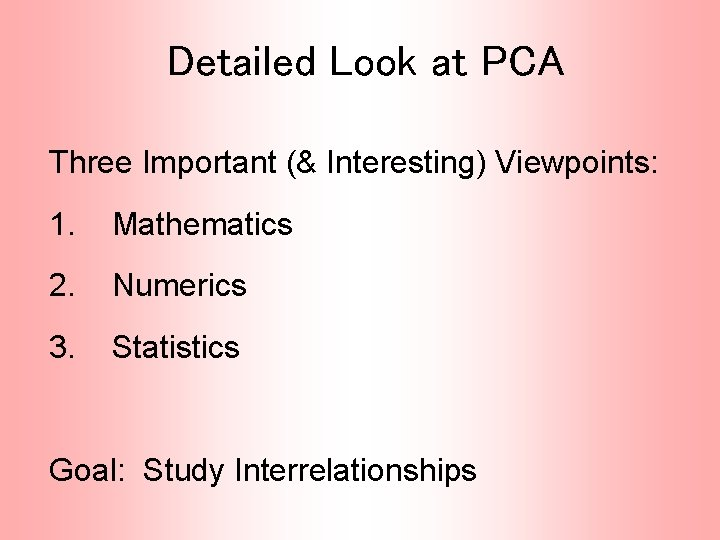Detailed Look at PCA Three Important (& Interesting) Viewpoints: 1. Mathematics 2. Numerics 3. Statistics Goal: Study Interrelationships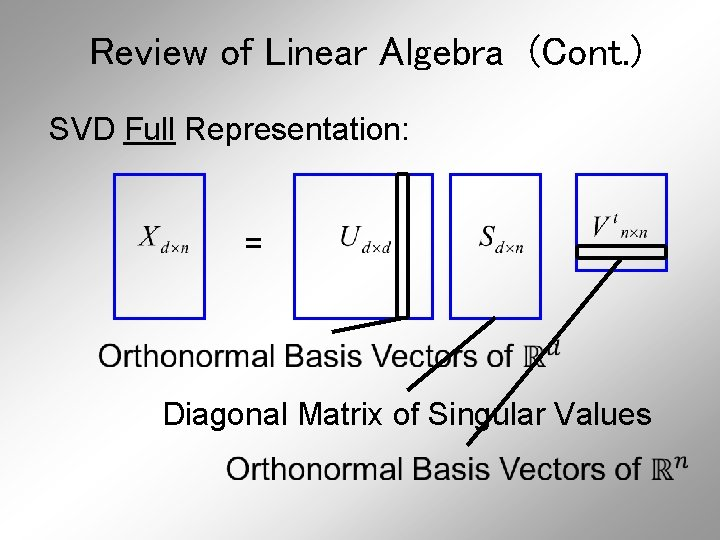Review of Linear Algebra (Cont. ) SVD Full Representation: = Diagonal Matrix of Singular Values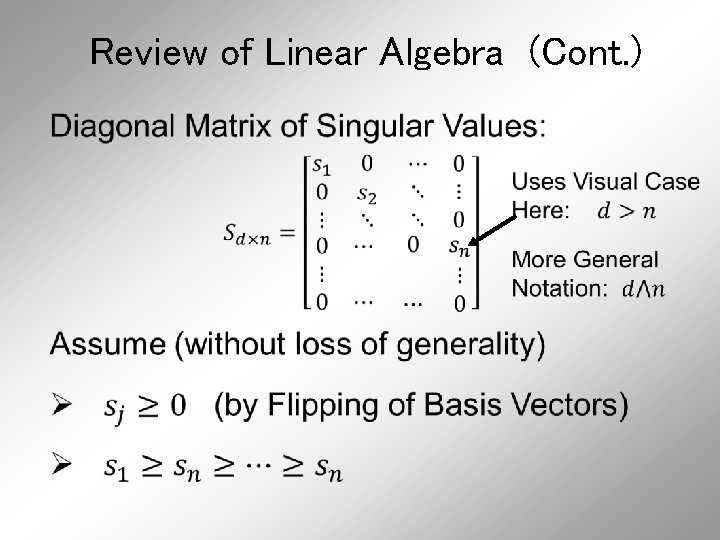Review of Linear Algebra (Cont. )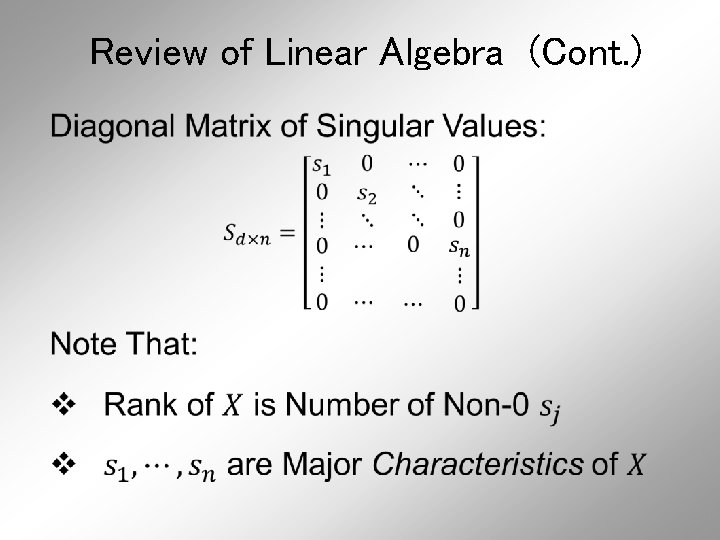Review of Linear Algebra (Cont. )Review of Linear Algebra (Cont. ) Isometry (~Rotation) Coordinate Rescaling Isometry (~Rotation)Covariance Matrices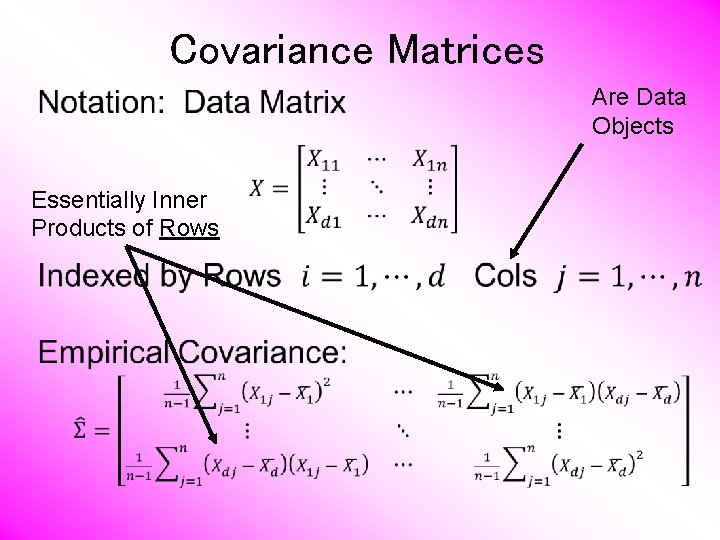Covariance Matrices Are Data Objects Essentially Inner Products of Rows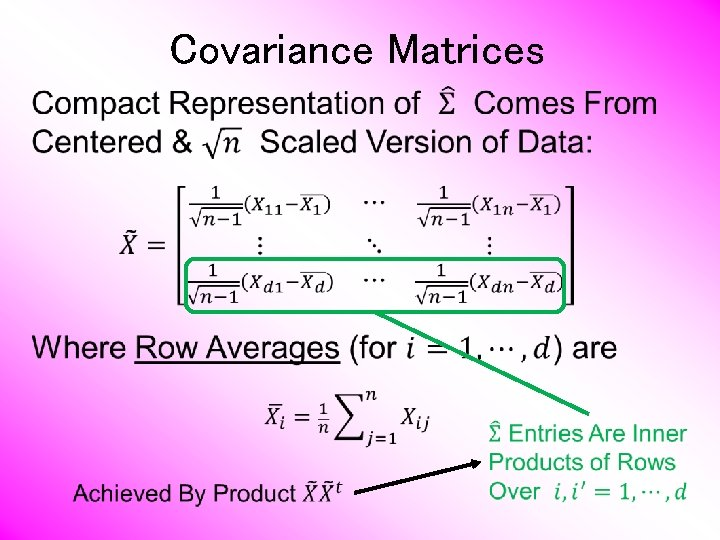Covariance MatricesCovariance Matrices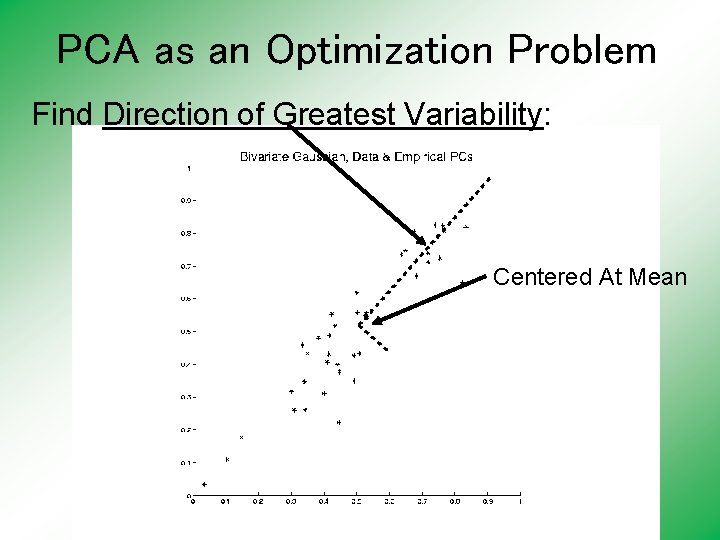PCA as an Optimization Problem Find Direction of Greatest Variability: Centered At Mean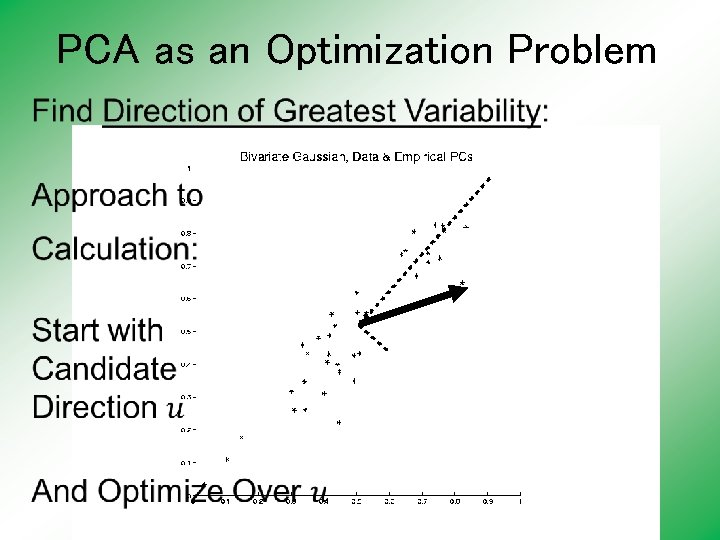PCA as an Optimization ProblemPCA as Optimization (Cont. ) Same Notation as AbovePCA as Optimization (Cont. ) Sample Variance of ScoresPCA as Optimization (Cont. ) Looks Like “Products Of Rows” From Above Cov. Matrix Repr’nPCA as Optimization (Cont. ) Diagonal Matrix of EigenvaluesPCA as Optimization (Cont. )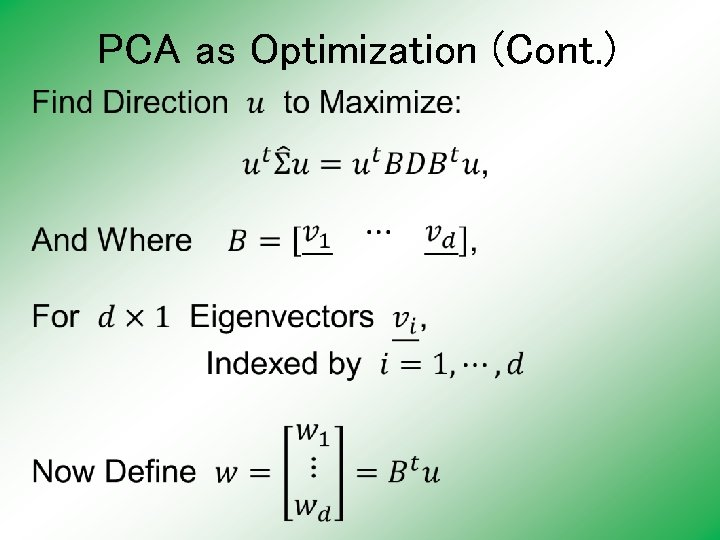PCA as Optimization (Cont. )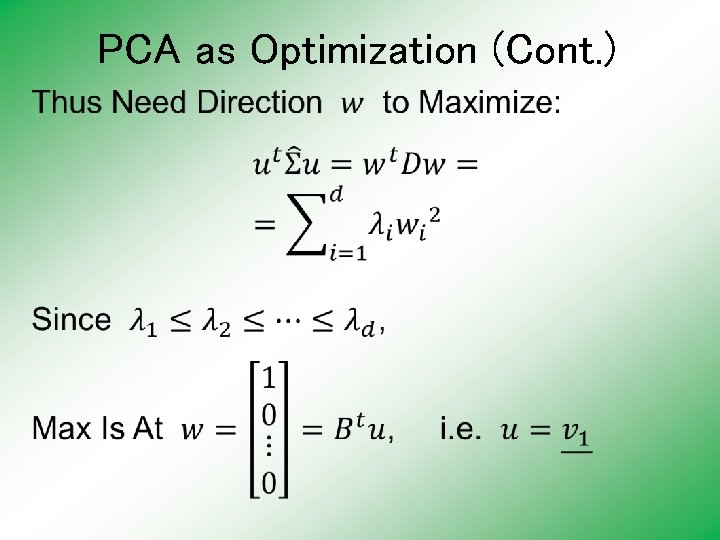PCA as Optimization (Cont. )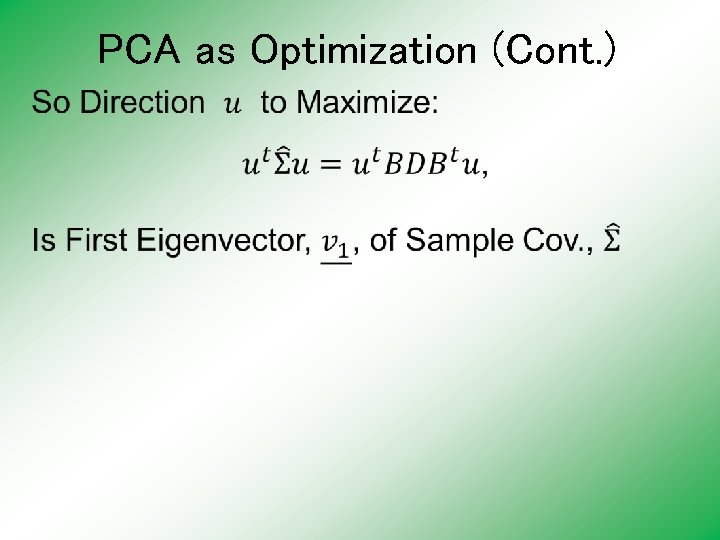PCA as Optimization (Cont. )PCA as Optimization (Cont. )PCA as Optimization (Cont. )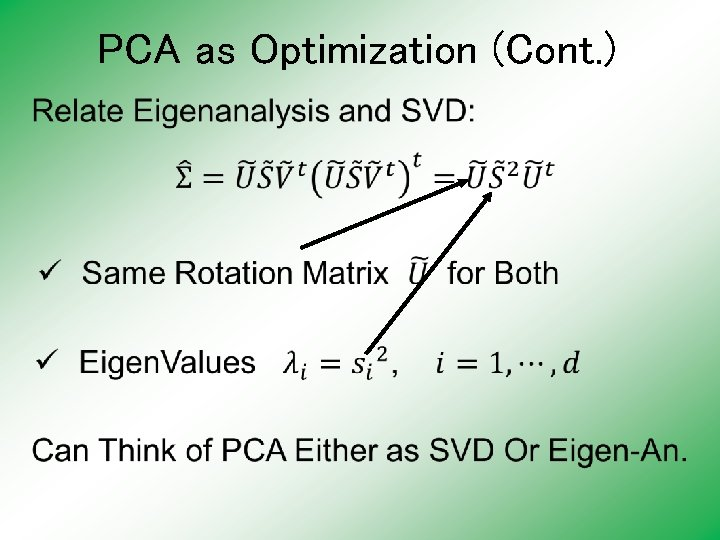PCA as Optimization (Cont. )PCA as Optimization (Cont. )PCA as Optimization (Cont. )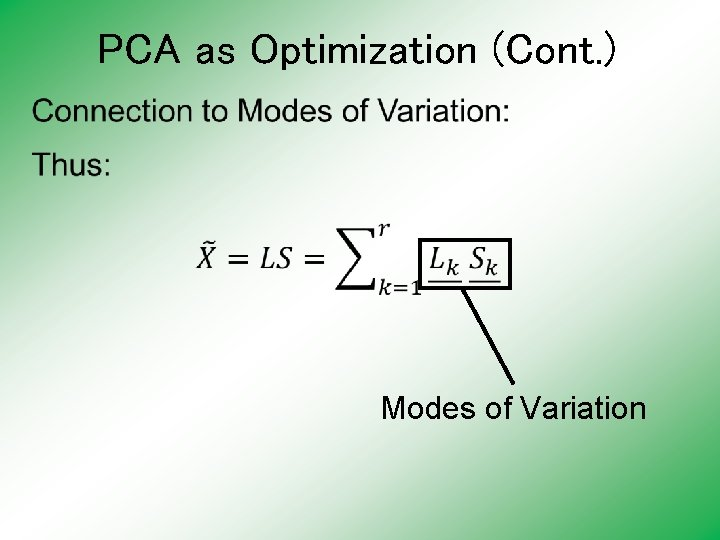PCA as Optimization (Cont. ) Modes of Variation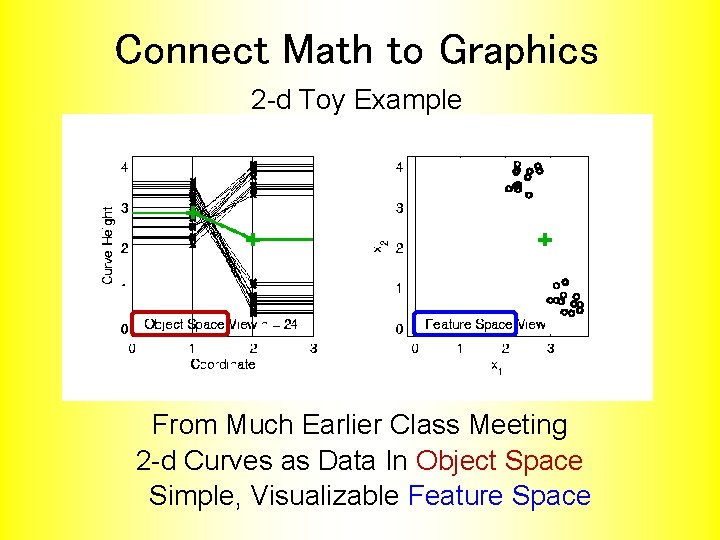Connect Math to Graphics 2 -d Toy Example From Much Earlier Class Meeting 2 -d Curves as Data In Object Space Simple, Visualizable Feature Space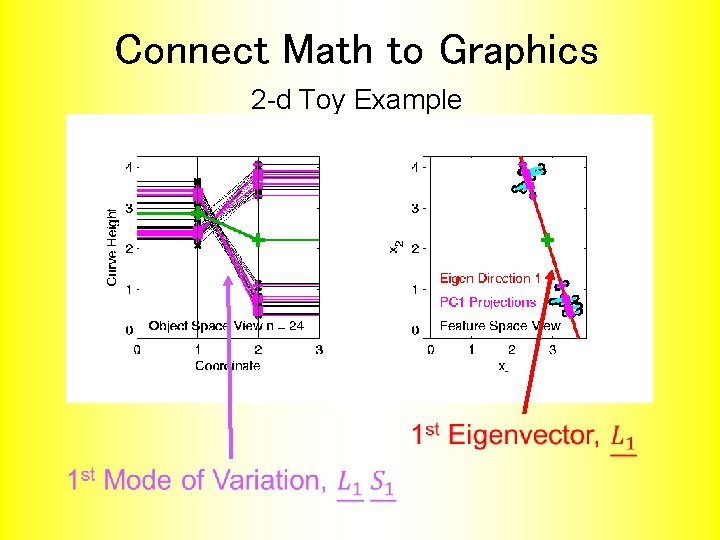Connect Math to Graphics 2 -d Toy ExampleConnect Math to Graphics 2 -d Toy ExamplePCA Redistribution of Energy Now for Scree Plots (Upper Right of FDA Anal. ) Carefully Look At: Ø Intuition Ø Relation to Eigenanalysis Ø Numerical CalculationPCA Redist’n of Energy (Cont. )PCA Redist’n of Energy (Cont. )PCA Redist’n of Energy (Cont. ) Note, have already considered some of these Useful Plots: • Power Spectrum (as %s) • Cumulative Power Spectrum (%) Recall Common Terminology: Power Spectrum is Called “Scree Plot” Kruskal (1964) Cattell (1966) (all but name “scree”) (1 st Appearance of name? ? ? )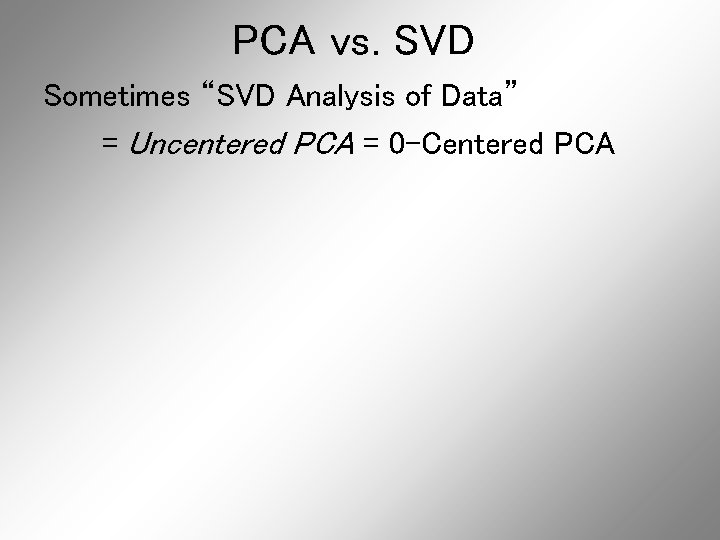PCA vs. SVD Sometimes “SVD Analysis of Data” = Uncentered PCA = 0 -Centered PCAPCA vs. SVD Sometimes “SVD Analysis of Data” = Uncentered PCA = 0 -Centered PCA Consequence: Skip this step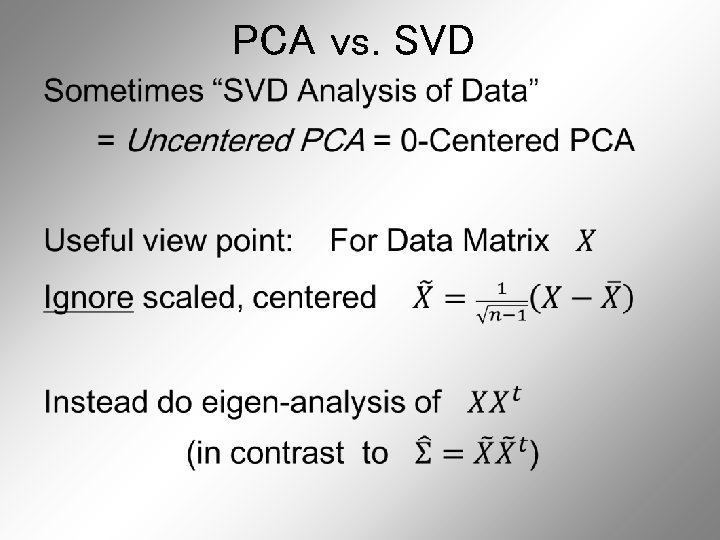PCA vs. SVD •PCA vs. SVD •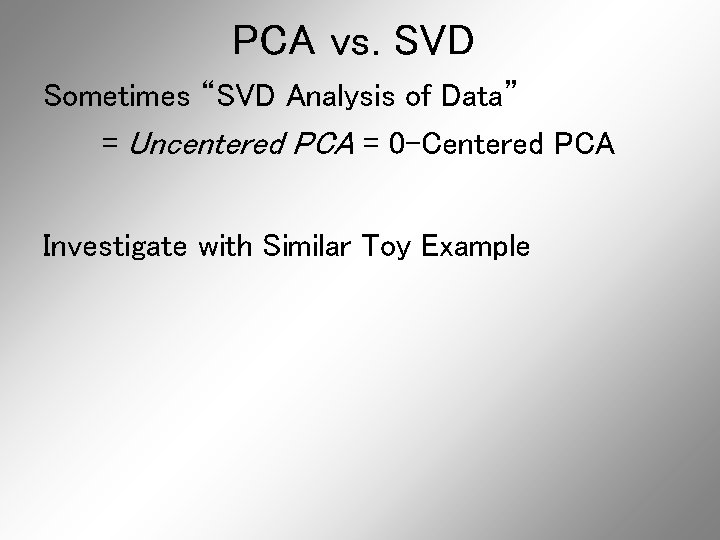PCA vs. SVD Sometimes “SVD Analysis of Data” = Uncentered PCA = 0 -Centered PCA Investigate with Similar Toy Example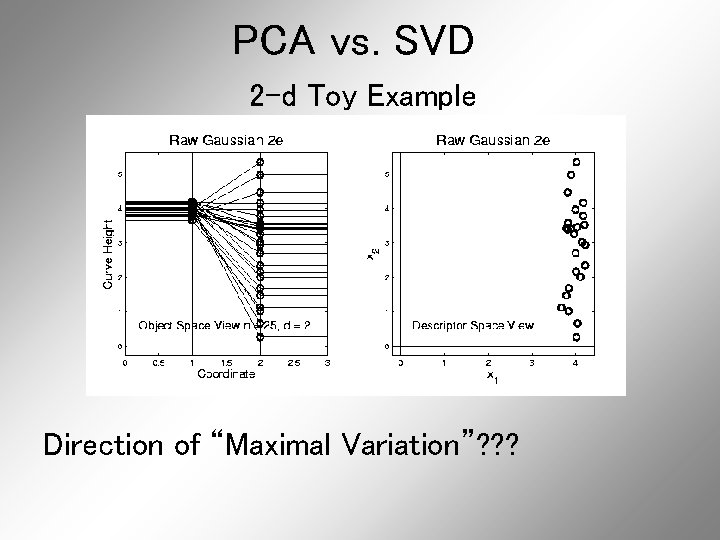PCA vs. SVD 2 -d Toy Example Direction of “Maximal Variation”? ? ?PCA vs. SVD 2 -d Toy Example Direction of “Maximal Variation”? ? ? PC 1 Solution (Mean Centered) Very Good!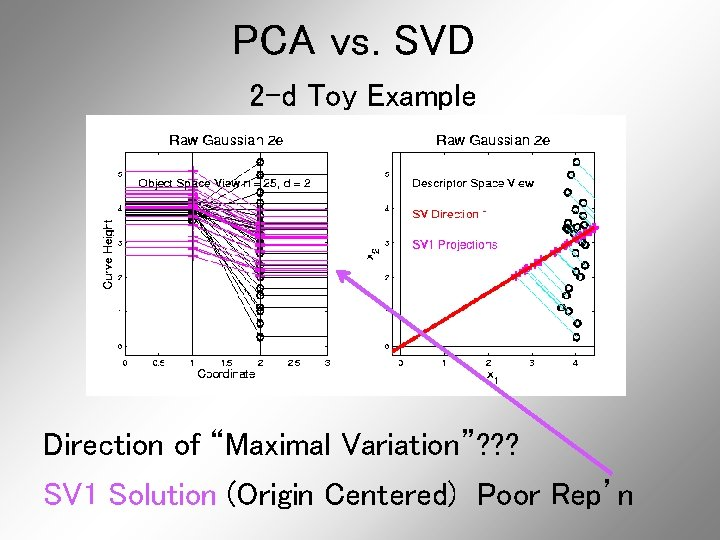PCA vs. SVD 2 -d Toy Example Direction of “Maximal Variation”? ? ? SV 1 Solution (Origin Centered) Poor Rep’nPCA vs. SVD 2 -d Toy Example Look in Orthogonal Direction: PC 2 Solution (Mean Centered) Very Good!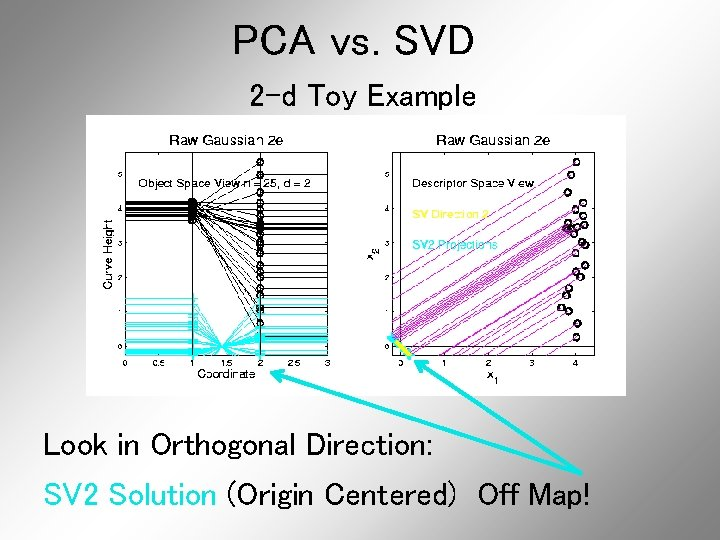PCA vs. SVD 2 -d Toy Example Look in Orthogonal Direction: SV 2 Solution (Origin Centered) Off Map!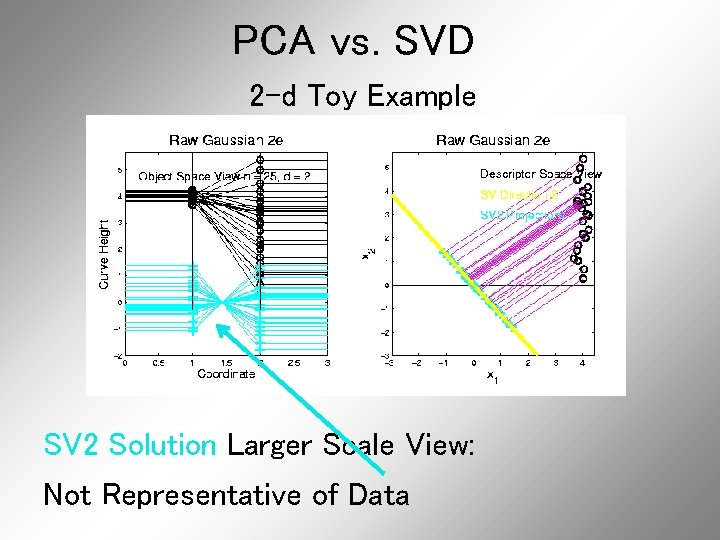PCA vs. SVD 2 -d Toy Example SV 2 Solution Larger Scale View: Not Representative of Data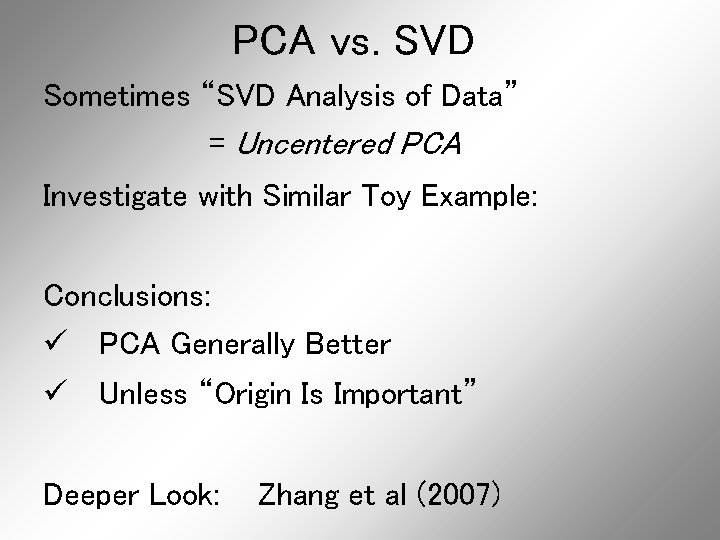PCA vs. SVD Sometimes “SVD Analysis of Data” = Uncentered PCA Investigate with Similar Toy Example: Conclusions: ü PCA Generally Better ü Unless “Origin Is Important” Deeper Look: Zhang et al (2007)Different Views of PCA Solves several optimization problems: 1. Direction to maximize SS of 1 -d proj’d data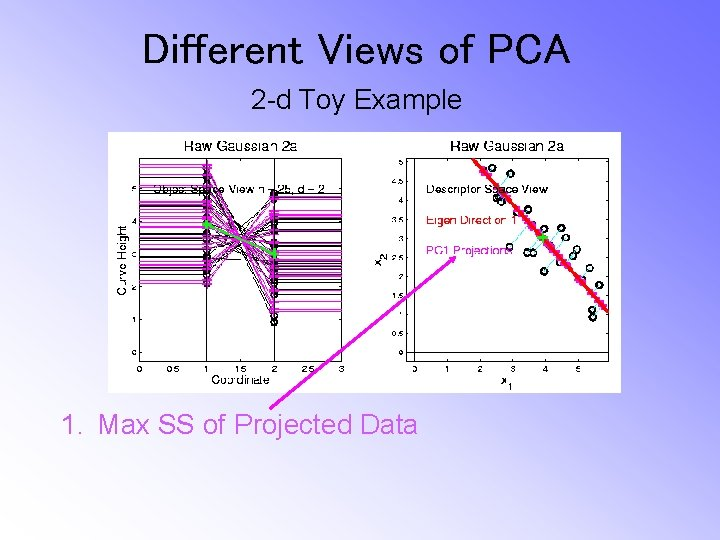Different Views of PCA 2 -d Toy Example 1. Max SS of Projected DataDifferent Views of PCA Solves several optimization problems: 1. Direction to maximize SS of 1 -d proj’d data 2. Direction to minimize SS of residuals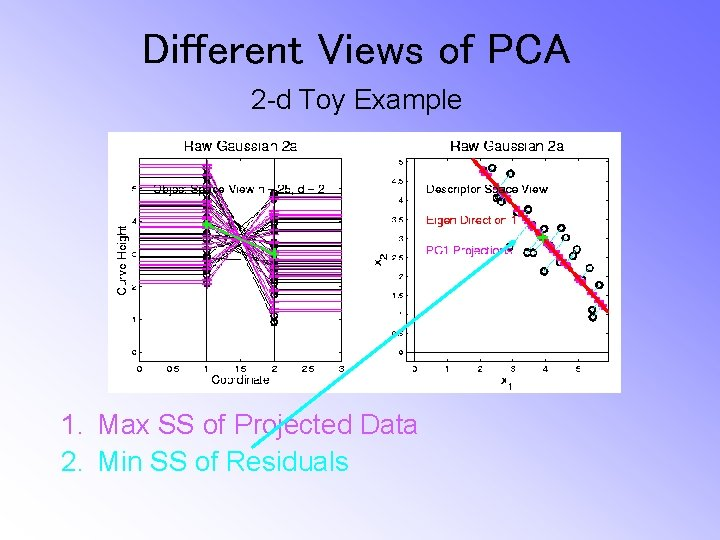Different Views of PCA 2 -d Toy Example 1. Max SS of Projected Data 2. Min SS of Residuals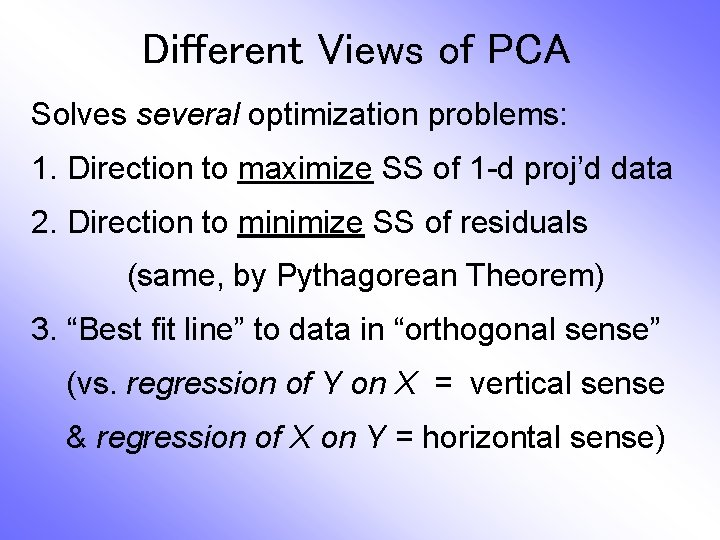Different Views of PCA Solves several optimization problems: 1. Direction to maximize SS of 1 -d proj’d data 2. Direction to minimize SS of residuals (same, by Pythagorean Theorem) 3. “Best fit line” to data in “orthogonal sense” (vs. regression of Y on X = vertical sense & regression of X on Y = horizontal sense)Different Views of PCA 2 -d Toy Example 1. Max SS of Projected Data 2. Min SS of Residuals 3. Best Fit LineDifferent Views of PCA Toy Example Comparison of Fit Lines: Ø PC 1 Ø Regression of Y on X Ø Regression of X on Y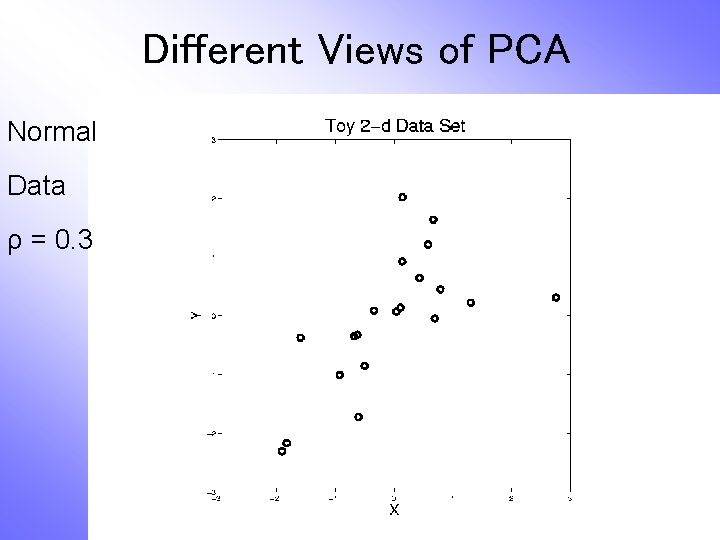Different Views of PCA Normal Data ρ = 0. 3Different Views of PCA Projected Residuals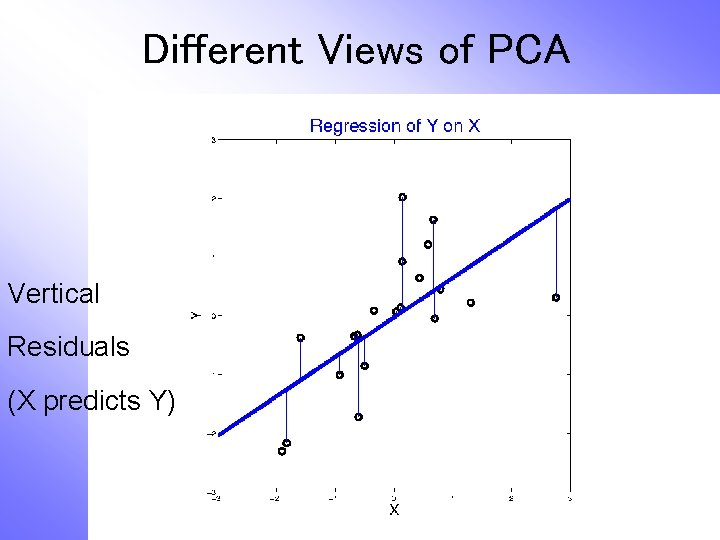Different Views of PCA Vertical Residuals (X predicts Y)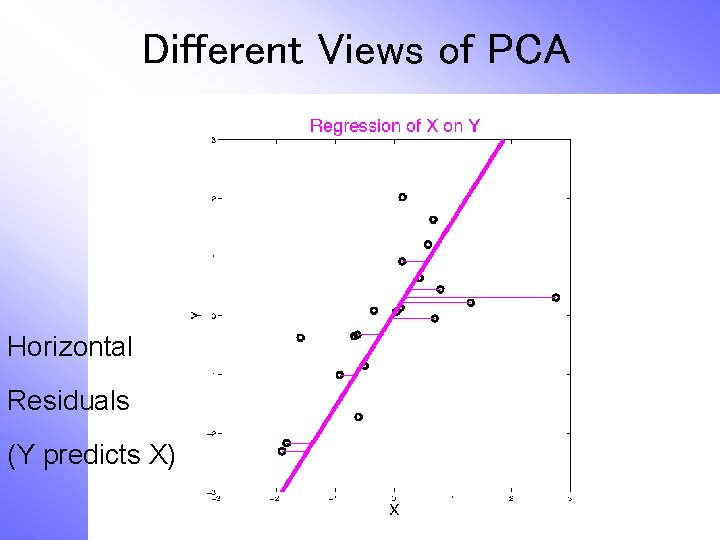Different Views of PCA Horizontal Residuals (Y predicts X)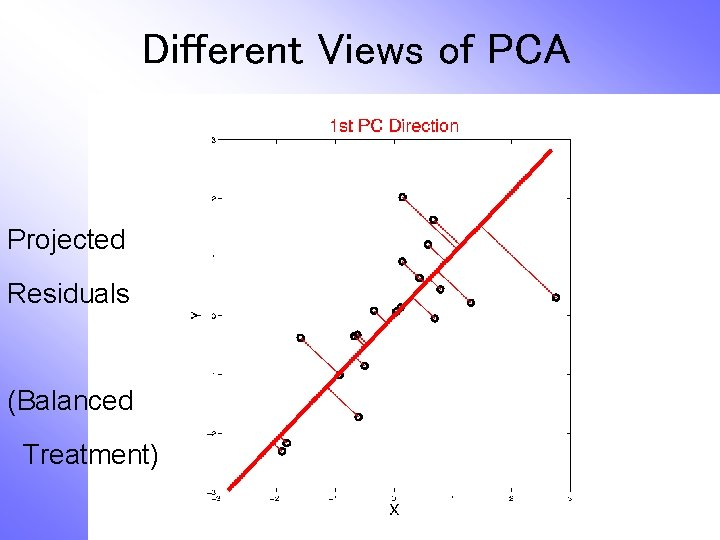Different Views of PCA Projected Residuals (Balanced Treatment)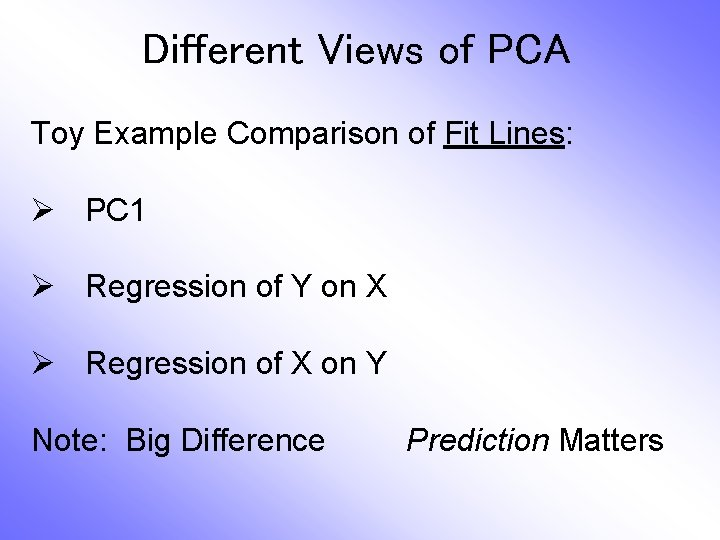Different Views of PCA Toy Example Comparison of Fit Lines: Ø PC 1 Ø Regression of Y on X Ø Regression of X on Y Note: Big Difference Prediction MattersDifferent Views of PCA Solves several optimization problems: 1. Direction to maximize SS of 1 -d proj’d data 2. Direction to minimize SS of residuals (same, by Pythagorean Theorem) 3. “Best fit line” to data in “orthogonal sense” (vs. regression of Y on X = vertical sense & regression of X on Y = horizontal sense) Use one that makes sense…Participant Presentation Siyao Liu: Clustering Single Cell RNAseq Data